# Lect 4 analog meter PMMC.pptx

27 de May de 2023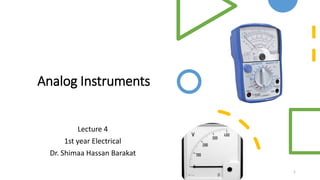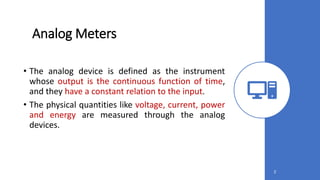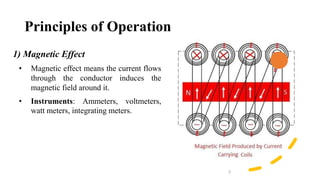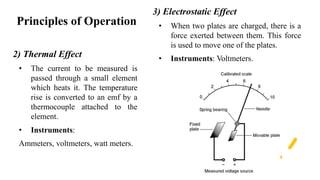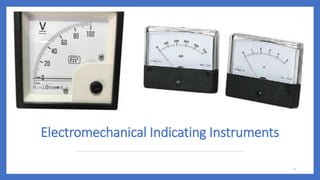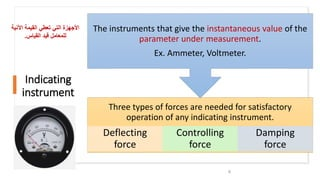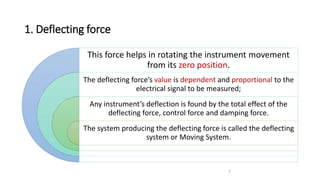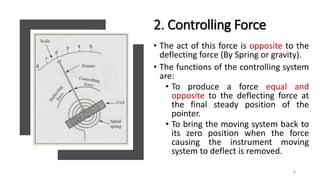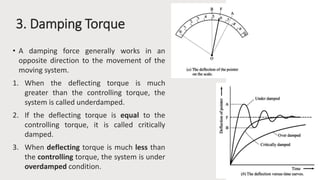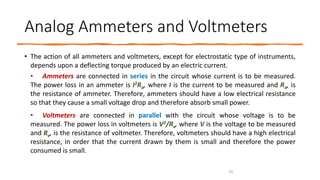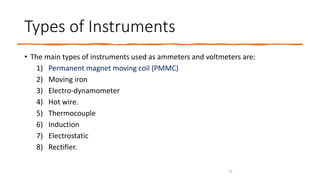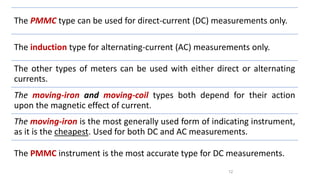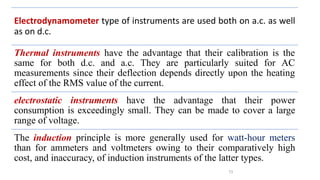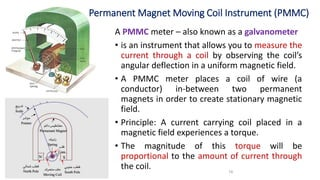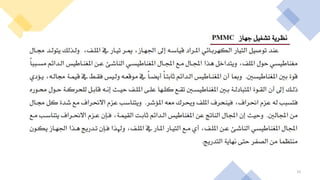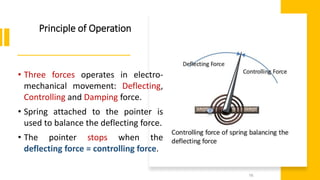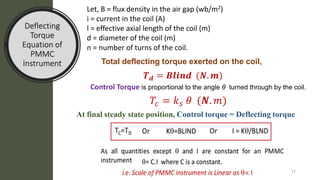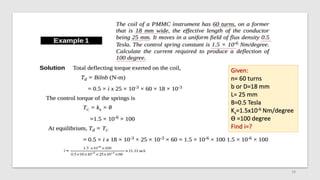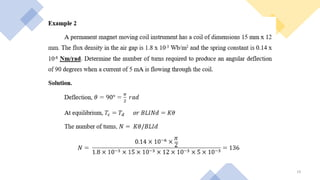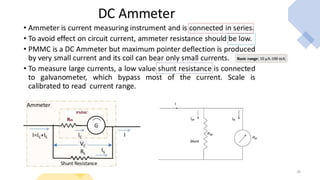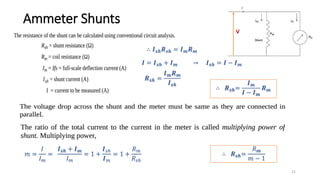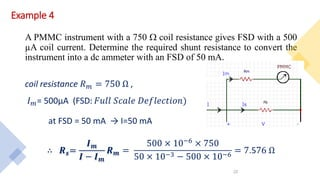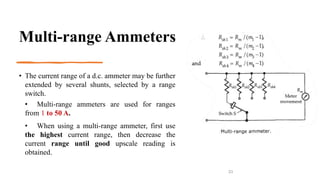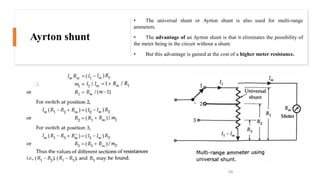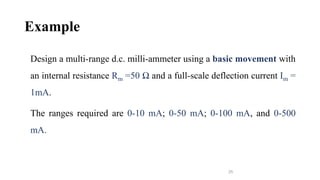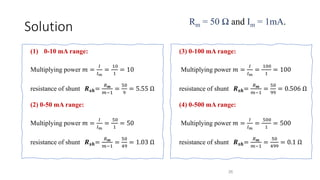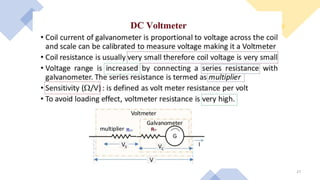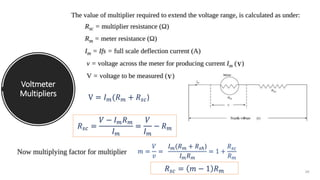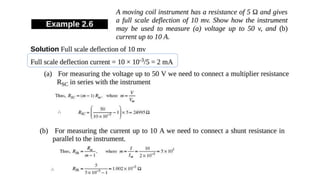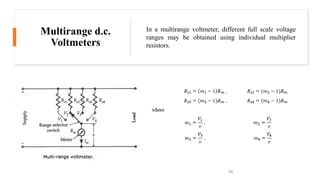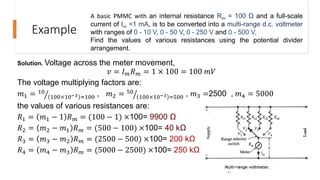1 de 31

### Lect 4 analog meter PMMC.pptx

• 1. Analog Instruments Lecture 4 1st year Electrical Dr. Shimaa Hassan Barakat 1
• 2. Analog Meters • The analog device is defined as the instrument whose output is the continuous function of time, and they have a constant relation to the input. • The physical quantities like voltage, current, power and energy are measured through the analog devices. 2
• 3. Principles of Operation 1) Magnetic Effect • Magnetic effect means the current flows through the conductor induces the magnetic field around it. • Instruments: Ammeters, voltmeters, watt meters, integrating meters. 3
• 4. Principles of Operation 2) Thermal Effect • The current to be measured is passed through a small element which heats it. The temperature rise is converted to an emf by a thermocouple attached to the element. • Instruments: Ammeters, voltmeters, watt meters. 4 3) Electrostatic Effect • When two plates are charged, there is a force exerted between them. This force is used to move one of the plates. • Instruments: Voltmeters.
• 5. Electromechanical Indicating Instruments 5
• 6. Indicating instrument 6 Three types of forces are needed for satisfactory operation of any indicating instrument. Deflecting force Controlling force Damping force The instruments that give the instantaneous value of the parameter under measurement. Ex. Ammeter, Voltmeter. ‫اآلني‬ ‫القيمة‬ ‫تعطي‬ ‫التي‬ ‫األجهزة‬ ‫ة‬ ‫القياس‬ ‫قيد‬ ‫للمعامل‬ .
• 7. 1. Deflecting force 7 This force helps in rotating the instrument movement from its zero position. The deflecting force’s value is dependent and proportional to the electrical signal to be measured; Any instrument’s deflection is found by the total effect of the deflecting force, control force and damping force. The system producing the deflecting force is called the deflecting system or Moving System.
• 8. 2. Controlling Force • The act of this force is opposite to the deflecting force (By Spring or gravity). • The functions of the controlling system are: • To produce a force equal and opposite to the deflecting force at the final steady position of the pointer. • To bring the moving system back to its zero position when the force causing the instrument moving system to deflect is removed. 8
• 9. 3. Damping Torque • A damping force generally works in an opposite direction to the movement of the moving system. 1. When the deflecting torque is much greater than the controlling torque, the system is called underdamped. 2. If the deflecting torque is equal to the controlling torque, it is called critically damped. 3. When deflecting torque is much less than the controlling torque, the system is under overdamped condition. 9
• 10. Analog Ammeters and Voltmeters • The action of all ammeters and voltmeters, except for electrostatic type of instruments, depends upon a deflecting torque produced by an electric current. • Ammeters are connected in series in the circuit whose current is to be measured. The power loss in an ammeter is I2Ra, where I is the current to be measured and Ra, is the resistance of ammeter. Therefore, ammeters should have a low electrical resistance so that they cause a small voltage drop and therefore absorb small power. • Voltmeters are connected in parallel with the circuit whose voltage is to be measured. The power loss in voltmeters is V2/Rv, where V is the voltage to be measured and Rv, is the resistance of voltmeter. Therefore, voltmeters should have a high electrical resistance, in order that the current drawn by them is small and therefore the power consumed is small. 10
• 11. Types of Instruments • The main types of instruments used as ammeters and voltmeters are: 1) Permanent magnet moving coil (PMMC) 2) Moving iron 3) Electro-dynamometer 4) Hot wire. 5) Thermocouple 6) Induction 7) Electrostatic 8) Rectifier. 11
• 12. The PMMC type can be used for direct-current (DC) measurements only. The induction type for alternating-current (AC) measurements only. The other types of meters can be used with either direct or alternating currents. The moving-iron and moving-coil types both depend for their action upon the magnetic effect of current. The moving-iron is the most generally used form of indicating instrument, as it is the cheapest. Used for both DC and AC measurements. The PMMC instrument is the most accurate type for DC measurements. 12
• 13. Electrodynamometer type of instruments are used both on a.c. as well as on d.c. Thermal instruments have the advantage that their calibration is the same for both d.c. and a.c. They are particularly suited for AC measurements since their deflection depends directly upon the heating effect of the RMS value of the current. electrostatic instruments have the advantage that their power consumption is exceedingly small. They can be made to cover a large range of voltage. The induction principle is more generally used for watt-hour meters than for ammeters and voltmeters owing to their comparatively high cost, and inaccuracy, of induction instruments of the latter types. 13
• 14. 14 A PMMC meter – also known as a galvanometer • is an instrument that allows you to measure the current through a coil by observing the coil’s angular deflection in a uniform magnetic field. • A PMMC meter places a coil of wire (a conductor) in-between two permanent magnets in order to create stationary magnetic field. • Principle: A current carrying coil placed in a magnetic field experiences a torque. • The magnitude of this torque will be proportional to the amount of current through the coil. Permanent Magnet Moving Coil Instrument (PMMC)
• 15. 15
• 16. Principle of Operation 16 • Three forces operates in electro- mechanical movement: Deflecting, Controlling and Damping force. • Spring attached to the pointer is used to balance the deflecting force. • The pointer stops when the deflecting force = controlling force.
• 17. Deflecting Torque Equation of PMMC Instrument 17 Let, B = flux density in the air gap (wb/m2) i = current in the coil (A) l = effective axial length of the coil (m) d = diameter of the coil (m) n = number of turns of the coil. Total deflecting torque exerted on the coil, 𝑻𝒅 = 𝑩𝒍𝒊𝒏𝒅 (𝑵. 𝒎) Control Torque is proportional to the angle θ turned through by the coil. 𝑇𝑐 = 𝑘𝑠 𝜃 (𝑵. 𝑚) At final steady state position, Control torque = Deflecting torque
• 18. 18 Given: n= 60 turns b or D=18 mm L= 25 mm B=0.5 Tesla Ks=1.5x10-6 Nm/degree ϴ =100 degree Find i=?
• 19. 19
• 20. 20
• 21. Ammeter Shunts 21 ∴ 𝑰𝒔𝒉𝑹𝒔𝒉 = 𝑰𝒎𝑹𝒎 𝑰 = 𝑰𝒔𝒉 + 𝑰𝒎 → 𝑰𝒔𝒉 = 𝑰 − 𝑰𝒎 𝑹𝒔𝒉 = 𝑰𝒎𝑹𝒎 𝑰𝒔𝒉 ∴ 𝑹𝒔𝒉= 𝑰𝒎 𝑰 − 𝑰𝒎 𝑹𝒎 𝑚 = 𝐼 𝐼𝑚 = 𝑰𝒔𝒉 + 𝑰𝒎 𝐼𝑚 = 1 + 𝑰𝑠ℎ 𝑰𝑚 = 1 + 𝑅𝑚 𝑅𝑠ℎ ∴ 𝑹𝒔𝒉= 𝑅𝒎 𝑚 − 1 V
• 22. Example 4 A PMMC instrument with a 750  coil resistance gives FSD with a 500 µA coil current. Determine the required shunt resistance to convert the instrument into a dc ammeter with an FSD of 50 mA. coil resistance 𝑅𝑚 = 750 Ω , 𝐼𝑚= 500μA (FSD: 𝐹𝑢𝑙𝑙 𝑆𝑐𝑎𝑙𝑒 𝐷𝑒𝑓𝑙𝑒𝑐𝑡𝑖𝑜𝑛) at FSD = 50 mA → I=50 mA ∴ 𝑹𝒔= 𝑰𝒎 𝑰 − 𝑰𝒎 𝑹𝒎 = 500 × 10−6 × 750 50 × 10−3 − 500 × 10−6 = 7.576 Ω 22
• 23. Multi-range Ammeters • The current range of a d.c. ammeter may be further extended by several shunts, selected by a range switch. • Multi-range ammeters are used for ranges from 1 to 50 A. • When using a multi-range ammeter, first use the highest current range, then decrease the current range until good upscale reading is obtained. 23
• 24. Ayrton shunt • The universal shunt or Ayrton shunt is also used for multi-range ammeters. • The advantage of an Ayrton shunt is that it eliminates the possibility of the meter being in the circuit without a shunt. • But this advantage is gained at the cost of a higher meter resistance. 24
• 25. Example Design a multi-range d.c. milli-ammeter using a basic movement with an internal resistance Rm =50 Ω and a full-scale deflection current Im = 1mA. The ranges required are 0-10 mA; 0-50 mA; 0-100 mA, and 0-500 mA. 25
• 26. Solution (1) 0-10 mA range: Multiplying power 𝑚 = 𝐼 𝐼𝑚 = 10 1 = 10 resistance of shunt 𝑹𝒔𝒉= 𝑅𝒎 𝑚−1 = 50 9 = 5.55 Ω (2) 0-50 mA range: Multiplying power 𝑚 = 𝐼 𝐼𝑚 = 50 1 = 50 resistance of shunt 𝑹𝒔𝒉= 𝑅𝒎 𝑚−1 = 50 49 = 1.03 Ω 26 (3) 0-100 mA range: Multiplying power 𝑚 = 𝐼 𝐼𝑚 = 100 1 = 100 resistance of shunt 𝑹𝒔𝒉= 𝑅𝒎 𝑚−1 = 50 99 = 0.506 Ω (4) 0-500 mA range: Multiplying power 𝑚 = 𝐼 𝐼𝑚 = 500 1 = 500 resistance of shunt 𝑹𝒔𝒉= 𝑅𝒎 𝑚−1 = 50 499 = 0.1 Ω Rm = 50 Ω and Im = 1mA.
• 27. 27
• 28. 28 Voltmeter Multipliers V = 𝐼𝑚(𝑅𝑚 + 𝑅𝑠𝑐) 𝑅𝑠𝑐 = 𝑉 − 𝐼𝑚𝑅𝑚 𝐼𝑚 = 𝑉 𝐼𝑚 − 𝑅𝑚 𝑅𝑠𝑐 = 𝑚 − 1 𝑅𝑚 𝑚 = 𝑉 𝑣 = 𝐼𝑚(𝑅𝑚 + 𝑅𝑠ℎ) 𝐼𝑚𝑅𝑚 = 1 + 𝑅𝑠𝑐 𝑅𝑚
• 29. 29
• 30. Multirange d.c. Voltmeters In a multirange voltmeter, different full scale voltage ranges may be obtained using individual multiplier resistors. 30
• 31. Example Solution. Voltage across the meter movement, 𝑣 = 𝐼𝑚𝑅𝑚 = 1 × 100 = 100 𝑚𝑉 The voltage multiplying factors are: 𝑚1 = 10 100×10−3 =100 , 𝑚2 = 50 100×10−3 =500 , 𝑚3 =2500 , 𝑚4 = 5000 the values of various resistances are: 𝑅1 = 𝑚1 − 1 𝑅𝑚 = (100 − 1) ×100= 9900 Ω 𝑅2 = 𝑚2 − 𝑚1 𝑅𝑚 = (500 − 100) ×100= 40 kΩ 𝑅3 = 𝑚3 − 𝑚2 𝑅𝑚 = (2500 − 500) ×100= 200 kΩ 𝑅4 = 𝑚4 − 𝑚3 𝑅𝑚 = (5000 − 2500) ×100= 250 kΩ 31 A basic PMMC with an internal resistance Rm = 100 Ω and a full-scale current of Im =1 mA, is to be converted into a multi-range d.c. voltmeter with ranges of 0 - 10 V, 0 - 50 V, 0 - 250 V and 0 - 500 V. Find the values of various resistances using the potential divider arrangement.